Saltar al contenido principal

# 8.7: Problems for Chapter 8

P8.1: Rank the following molecules in order of how fast they would be expected to react with CH3SNa in acetone:P8.2: Draw line structures representing the most stable carbocation with the given molecular formula:

a) C3H7+

b) C4H9+

c) C3H8N+

d) C4H7+

P8.3:  Which of the two carbocations below is expected to be lower in energy?  Explain your reasoning.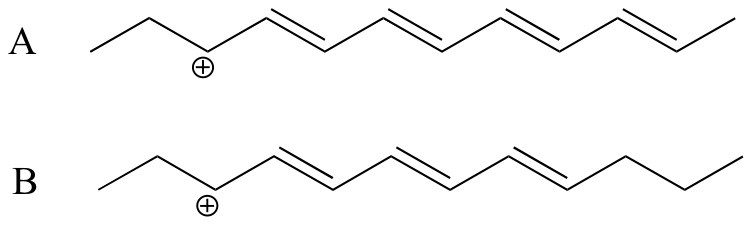P8.4: Arrange the following species in order of increasing nucleophilicity.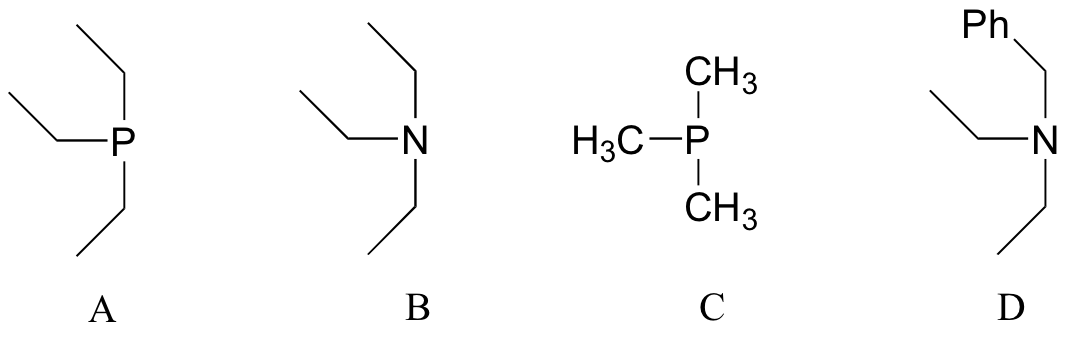P8.5: Predict the organic products of the following SN2 reactions. Indicate stereochemistry.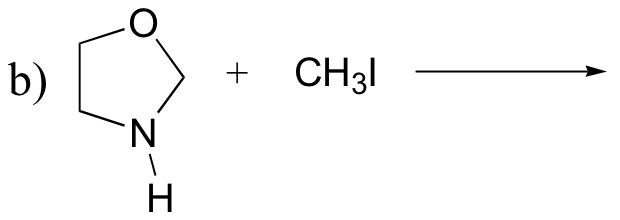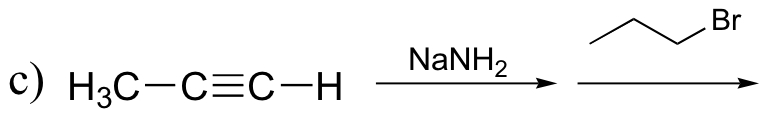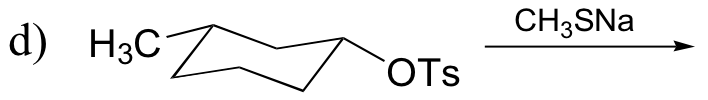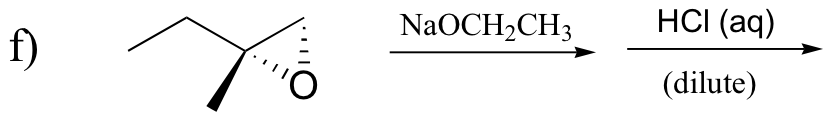P8.6: Which of the SN2 reactions in the previous problem is likely to have a first order rate expression?   Explain.

P8.7:  From the following pairs, select the better nucleophile:

a) water or hydroxide ion

b) CH3S- or CH3OH

c) CH2S- or CH3SH

d) acetate ion  or hydroxide ion

e) diethyl sulfide or diethyl ether

f) dimethylamine or diethylether

g) trimethylamine or 2,2-dimethylpropane

P8.8: Methyl iodide (0.10 mole) is added to a solution that contains 0.10 mole NaOCH3 and 0.10 mole NaSCH3 in ethanol solvent.  Predict the major product that would be isolated, and explain your reasoning.

P8.9:  For each pair of compounds, predict which will more rapidly undergo SN1 solvolysis in a water/methanol solvent.P8.10: Predict the solvolysis products (considering stereochemistry) of each of the reactions below. Show the carbocation intermediate in each reaction.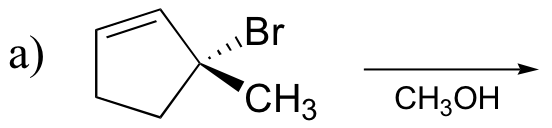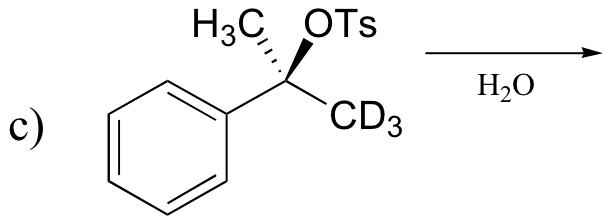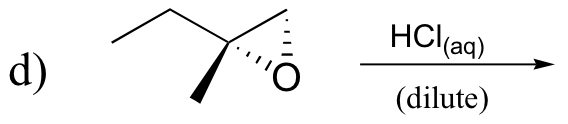P8.11: The following compounds are all direct products of nucleophilic substitution reactions.  Show the starting compounds.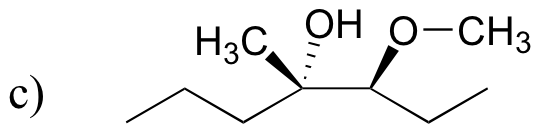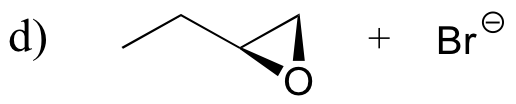P8.12: The following is the final step in the formation of a ‘disulfide bond’ between two cysteine residues within a protein (we’ll learn more about disulfide bonds in section 16.12A).  Propose a mechanism for this process, using H-A and :B to represent catalytic acid and base groups. Label those atoms which are acting as nucleophile, electrophile, and leaving group, respectively.P8.13: The figure below shows the regeneration of the disulfide bond in a coenzyme called lipoamide (we’ll learn more about one very important metabolic role of  lipoamide in section 16.12B).  In the process, a disulfide bond in the enzyme is broken to release two free cysteines.  Propose a likely mechanism for this transformation (hint – there are two SN2 displacements involved).P8.14: The ‘bicyclo’ compound shown below is very unreactive in SN1 reactions, even though it is a tertiary alkyl halide.  Explain. (Hint – consider bond geometry - a model will be very helpful!)Challenge problems

C8.1:In a classic experiment in physical organic chemistry, (R)-2-iodooctane was allowed to react with a radioactive isotope of  iodide ion, and the researchers monitored  how fast the radioactive iodide was incorporated into the alkane (the rate of incorporation, ki ) and also how fast optical activity was lost (the rate of racemization, kr).  They found that the rate of racemization was, within experimental error, equal to twice the rate of incorporation.  Discuss the significance of this result - what does it say about the actual mechanism of the reaction?

Template:Soderberg

$$\newcommand{\vecs}{\overset { \rightharpoonup} {\mathbf{#1}} }$$ $$\newcommand{\vecd}{\overset{-\!-\!\rightharpoonup}{\vphantom{a}\smash {#1}}}$$$$\newcommand{\id}{\mathrm{id}}$$ $$\newcommand{\Span}{\mathrm{span}}$$ $$\newcommand{\kernel}{\mathrm{null}\,}$$ $$\newcommand{\range}{\mathrm{range}\,}$$ $$\newcommand{\RealPart}{\mathrm{Re}}$$ $$\newcommand{\ImaginaryPart}{\mathrm{Im}}$$ $$\newcommand{\Argument}{\mathrm{Arg}}$$ $$\newcommand{\norm}{\| #1 \|}$$ $$\newcommand{\inner}{\langle #1, #2 \rangle}$$ $$\newcommand{\Span}{\mathrm{span}}$$ $$\newcommand{\id}{\mathrm{id}}$$ $$\newcommand{\Span}{\mathrm{span}}$$ $$\newcommand{\kernel}{\mathrm{null}\,}$$ $$\newcommand{\range}{\mathrm{range}\,}$$ $$\newcommand{\RealPart}{\mathrm{Re}}$$ $$\newcommand{\ImaginaryPart}{\mathrm{Im}}$$ $$\newcommand{\Argument}{\mathrm{Arg}}$$ $$\newcommand{\norm}{\| #1 \|}$$ $$\newcommand{\inner}{\langle #1, #2 \rangle}$$ $$\newcommand{\Span}{\mathrm{span}}$$